The boundary-layer theory (3) -Comparison with numerical calculations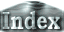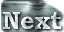Figure 8 shows a comparison between the boundary-layer theory and the numerical results. The solid red line is the averaged temperature obtained from equation (9) and the symbols show the results of the numerical calculations.. The two calculations are in good agreement, implying that the averaged temperature beneath the plate estimated by numerical experiments is well explained by the analytical formula. Figure 8(a) also reveals that the difference between the averaged temperature beneath and outside the plate gets bigger as the Rayleigh number increases. Figure 8(b) shows that the heat flux at the surface covered with a plate is less than that without a plate, and the difference between these two cases also becomes larger as the Rayleigh number increases. These characteristics are consistent with each other. Figure 8: Comparison between the results from the boundary-layer theory and the numerical calculations. d=0.1. Solid and broken lines show the estimates obtained using the boundary-layer theory for cases with and without a plate, respectively.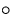and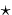denote the results of numerical calculations for cases with and without a plate, respectively. (a) The averaged temperature of fluid.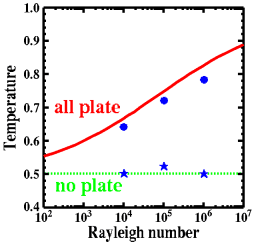(b) Surface heat flux (Nusselt number).

 The boundary-layer theory (3) -Comparison with numerical calculations